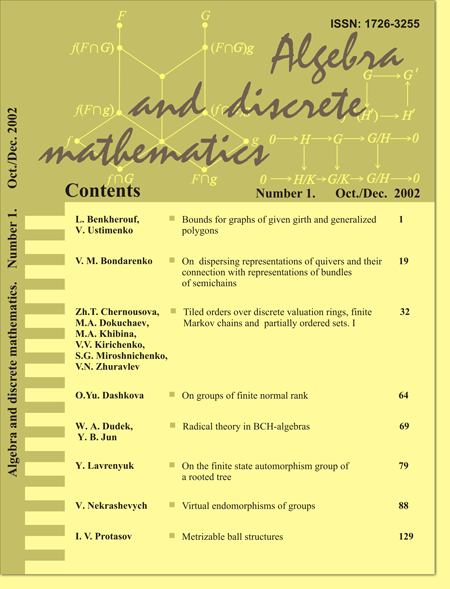The aim of the journal “Algebra and Discrete Mathematics” is to present timely the state-of-the-art accounts on modern research in all areas of algebra (general algebra, semigroups, groups, rings and modules, linear algebra, algebraic geometry, universal algebras, homological algebra etc.) and discrete mathematics (combinatorial analysis, graphs theory, mathematical logic, theory of automata, coding theory, cryptography etc.). Journal is sponsored by the Lugansk National Taras Shevchenko University with the science support of the Kyiv National Taras Shevchenko University. The papers published in Algebra and Discrete Mathematics are reviewed in Mathematical Reviews and Zentralblatt fur Mathematik Algebra and Discrete Mathematics has been indexed by Scopus since 2012 We encourage the authors to submit papers for the issues, see Instructions for authors